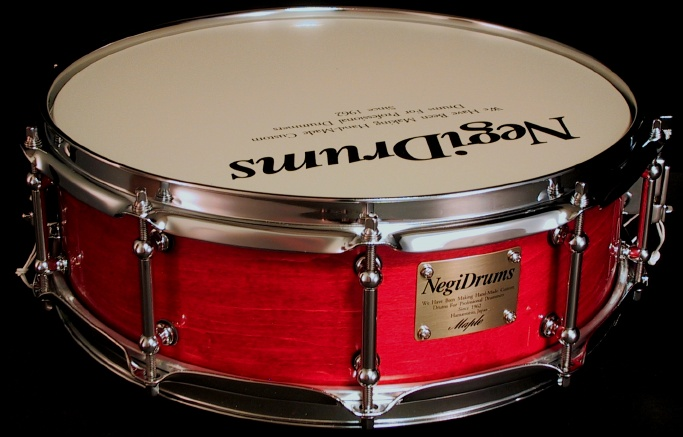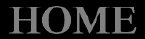\$BIJHV(B \$BDj2A(B \$B!JK\BN2A3J!K(B ND'sG\$BHNGd2A3J(B \$BIJHV(B \$BDj2A(B \$B!JK\BN2A3J!K(B ND'sG\$BHNGd2A3J(B \$BIJHV(B \$BDj2A(B \$B!JK\BN2A3J!K(B ND'sG\$BHNGd2A3J(B \$BIJHV(B \$BDj2A(B \$B!JK\BN2A3J!K(B ND'sG\$BHNGd2A3J(B \$BIJHV(B \$BDj2A(B \$B!JK\BN2A3J!K(B ND'sG\$BHNGd2A3J(B \$BIJHV(B \$BDj2A(B \$B!JK\BN2A3J!K(B ND'sG\$BHNGd2A3J(B (IOCX1Y(B \$B%5%\$%:(B (I6W0(B \$B%a%\$%W%k(B 8.0m/m (I6W0A,0D(B A B D E F G \$B%a%\$%W%k(B 8.0m/m (I6W0A,0D(B A B D E F G \$B%a%\$%W%k(B 8.0m/m (I6W0A,0D(B A B D E F G \$B%"%/%j%k(B 6.0m/m (I18XY8X10(B \$B%"%/%j%k(B 6.0m/m (I18XY8X10(B \$B%"%/%j%k(B 6.0m/m (I18XY8X10(B \$B%i%0(B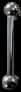\$B%/%i%7%+%k(B \$B%A%e!<%V%i%0(B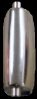\$B%O%\$%F%s%7%g%s(B \$B%i%0(B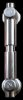\$B%o%\$%I(B \$B%A%e!<%V%i%0(B\$B%/%i%7%+%k(B \$B%A%e!<%V%i%0(B\$B%O%\$%F%s%7%g%s(B \$B%i%0(B\$B%O%\$%F%s%7%g%s(B \$B%i%0(B 14"*3.5" MU1435P \46,440 (\43,000) \41,796 NON NON AUI1435P \46,440 (\43,000) \41,796 NON NON 14"*4.0" MU1440P \50,760 (\47,000) \45,684 NON NON AU140P \50,760 (\47,000) \45,684 NON NON 14"*4.5" MU1445P \52,920 (\49,000) \47,628 MR1445P \52,920 (\49,000) \47,628 MK1445P \52,920 (\49,000) \47,628 AU1445P \52,920 (\49,000) \47,628 AR1445P \52,920 (\49,000) \47,628 AK1445P \52,920 (\49,000) \47,628 14"*5.0" MU1450P \54,000 (\50,000) \48,600 MR1450P \54,000 (\50,000) \48,600 MK1450P \54,000 (\50,000) \48,600 AU1450P \54,000 (\50,000) \48,600 AR1450P \54,000 (\50,000) \48,600 AK1450P \54,000 (\50,000) \48,600 14"*5.5" MU1455P \54,000 (\50,000) \48,600 MR1455P \54,000 (\50,000) \48,600 MK1455P \54,000 (\50,000) \48,600 AU1455P \54,000 (\50,000) \48,600 AR1455P \54,000 (\50,000) \48,600 AK1455P \54,000 (\50,000) \48,600 14"*6.0" MU1460P \55,080 (\51,000) \49,572 MR1460P \55,080 (\51,000) \49,572 MK1460P \55,080 (\51,000) \49,572 AU1460P \55,080 (\51,000) \49,572 AR1460P \55,080 (\51,000) \49,572 AK1460P \55,080 (\51,000) \49,572 14"*6.5" MU1465P \55,080 (\51,000) \49,572 MR1465P \55,080 (\51,000) \49,572 MK1465P \55,080 (\51,000) \49,572 AU1465P \55,080 (\51,000) \49,572 AR1465P \55,080 (\51,000) \49,572 AK1465P \55,080 (\51,000) \49,572 14"*7.0" MU1470P \56,160 (\52,000) \50,544 MR1470P \56,160 (\52,000) \50,544 MK1470P \56,160 (\52,000) \50,544 AU1470P \56,160 (\52,000) \50,544 AR1470P \56,160 (\52,000) \50,544 AK1470P \56,160 (\52,000) \50,544 14"*7.5" MU1475P \56,160 (\52,000) \50,544 MR1475P \56,160 (\52,000) \50,544 MK1475P \56,160 (\52,000) \50,544 AU1475P \56,160 (\52,000) \50,544 AR1475P \56,160 (\52,000) \50,544 AK1475P \56,160 (\52,000) \50,544 14"*8.0" MU1480P \57,240 (\53,000) \51,516 MR1480P \57,240 (\53,000) \51,516 MK1480P \57,240 (\53,000) \51,516 AU1480P \57,240 (\53,000) \51,516 AR1480P \57,240 (\53,000) \51,516 AK1480P \57,240 (\53,000) \51,516

\$B!!!!(B\$B!!(B Negi Drums

\$B!!!!!!!!!!!!!!%Q%o!<%U!<%W!!(B

\$B!!!!(B